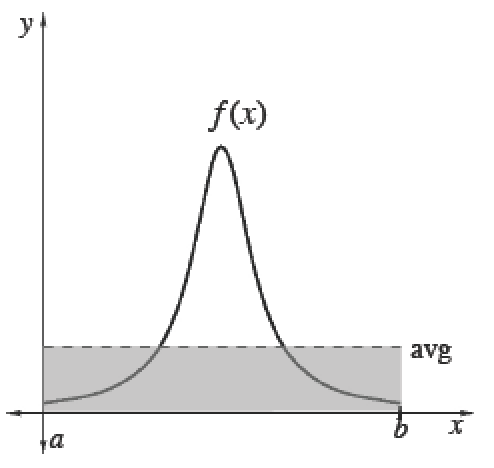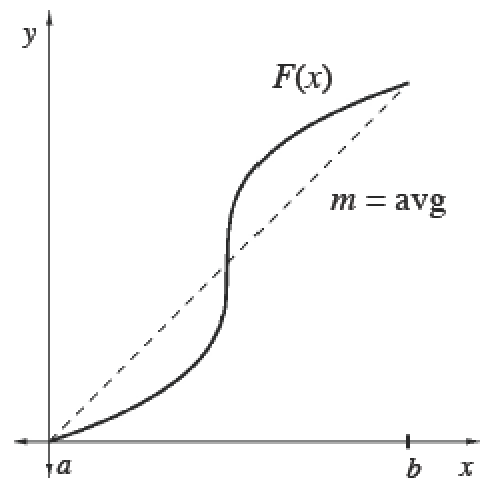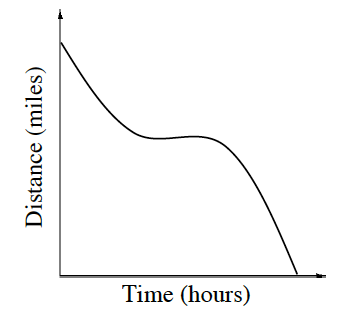### Home > APCALC > Chapter 7 > Lesson 7.3.2 > Problem7-115

7-115.

If the graph at right represents distance over time between a space shuttle and Earth, how would you determine the average velocity? Describe the method you would use.

Given a distance function, will average velocity be represented by 'average area under the curve' or 'average rate of change'?

Average (Mean) Values

 To calculate the mean (average) value of a finite set of items, add up the values of items and divide by the number of items.Integrals help us add over a continuous interval. Therefore, for any continuous function $f$ :$\frac{\int_a^bf(x)dx}{b-a}=$ mean value of $f$ over $[a, b]$Since $\int _ { a } ^ { b } f ( x ) d x$, we can also calculate the average value of any function $f$ using its antiderivative $F$. Its average slope gives the average rate of change of $F$, which is the same as the average value of $f.$$\frac{\int_a^bf(x)dx}{b-a}=\frac { F ( b ) - F ( a ) } { b - a }=$  mean rate of change of $F ′$ over $[a, b]$